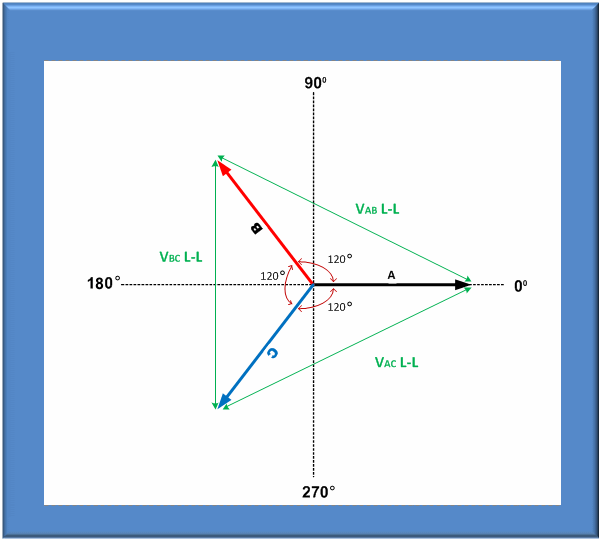Single Phase AC Voltage

Most of us are familiar with the single phase voltage in our homes provided by the local utility. For the US, this is typically 120V. For single phase voltage, the voltage is expressed as a Line to Neutral voltage between two power conductors (plus a safety ground).  The neutral conductor is normally at ground potential while the Line conductor is a sinusoidal AC voltage with an RMS value of 120Vac. That means the peak of the AC voltage alternates from +169.7Vac to -169.7Vac every 16.667 msec on the US 60Hz grid frequency. For many other countries, these nominal values are 230Vrms @ 50Hz (20 msec) instead.Figure 1: Single Phase 120Vrms Sinusoidal Voltage Waveform

Power Limited

Single phase voltage can deliver only so much power as all power has to be delivered using the line and neutral conductors. This is no problem for home use but for industrial use, more current may be required to run machines, motors, lighting and other high power loads.  For these situations, it is often desirable to raise both the voltage and the current to deliver this higher power. One option is to use two phase as found in some US home to run electric dryers.  This is called a split phase connection where two 120Vrms phases are 180° phase apart, providing twice the 120VLN or 240V Line-to-Line voltage.  This doubles the available power. Split phase is not commonly used in Europe or Asia as the normal single phase grid voltage is already 220VLN to 240LN.

Three Phase AC Voltage

Taking this one step further, high power loads are typically powered using three phases. This distributes the current over three instead of one set of wires, allowing for smaller and thus less expensive wiring.  The three voltage sources are phase shifted 120° with respect to each other to balance the load currents.  This is illustrated in Figure 2.Figure 2: Three Phase Voltage Waveforms with different rotations

The 120° phase shift between each waveform can be done in one of two phase rotations – A -> B -> C or A -> C -> B. Phase rotation does not affect most loads except for three phase AC motors which will turn in opposite direction if the phase rotation is changed.  Changing phase rotation can be accomplished by swapping any two of three phase connections.  When using a programmable AC power source like the AFX Series, phase angles for phase B and C can be programmed to either 120° and 240° or 240° and 120° respectively to change phase rotation.  The AFX also allows phase imbalances to be programmed to study the effect of phase variations on a unit under test.

Caution when Determining Line to Line Voltages

While the ‘normal’ three phase delta and wye voltage relationship is easily captured in a simple formula, this applies only with equal line to neutral voltages, perfect phase balance and sinusoidal voltages.  For this ideal case, the relationship between Line to Neutral RMS voltage and Line to Line RMS voltage can be expressed by the following formula:This relationship between line to neutral and line to line voltage is shown in the phase diagram of Figure 3.Figure 3: Three Phase Phasor Diagram

Figure 4 below shows two typical examples of three phase utility grid voltage configurations used in the United States.  Europe and Asia typically use 220/380V or 230/400V configurations instead.  The 120VLN per phase is equivalent with the 208VLL vector sum:

VLL = 120VLN * 1.732 = 207.84VLL

Note that the 480V delta grid configuration has no neutral connection and is referred to as a 3Wire + Ground Delta connection. To simulate this type of grid with an AC power source, the 3 phase load is connected as a delta between the three output phases only with no connection to the neutral output terminal.Figure 4: Typical Three Phase Voltage Configurations used in the USA

This ratio of √3 is important when using a programmable three phase AC power source as all T&M style AC power sources are only programmable in Line to Neutral voltage.  Thus, if any of the stated conditions are not true, you cannot just rely on this formula to determine Line to Line voltage:

1. Identical VLN voltages on all three phases
2. Balanced Phase angles on phase B and C
3. Low distortion, pure sinewave

A small phase shift on one or more of the three phases can have a significant impact on VLL voltages which results in load current imbalance as well.

A distorted voltage caused by a non-linear load on one of more phases can also throw off the Line to Line voltages.

Why Does it Matter?

Programmable three phase AC power sources have adjustable phase angles and often support arbitrary waveform capability. That means the relationship between line to neutral and line to line voltage is not necessarily ‘fixed’.  As a rule, all three phase programmable AC power sources are programmed in Line to Neutral RMS, regardless of the load type (Delta or Wye).  As such, it may be necessary to actually measure the resulting Line to Line voltage as calculating it is not valid if these conditions are not met.

Conclusion

When testing three phase loads, pay close attention to voltage and phase parameters when making assumptions about the Line to Line voltages applied to the unit under test.